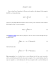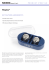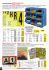# Physics 112 Sample Test 2 NAME __________________________

## Transcription

Physics 112 Sample Test 2 NAME __________________________
```Physics 112 Sample Test 2
NAME __________________________
Lorentz Force (single charge)
Lorentz Force (current element)
Force on a current carrying wire of length L
Magnetic Moment
Torque on a current Loop
Biot-Savart Law
Ampere’s Law
Infinite wire
Definition of Magnetic flux
Magnetic field due to:
Arc of current
Loop of current on central axis
Physics 112 Sample Test 2
NAME __________________________
Lorentz Force (single charge)
⃑
⃑⃑
Lorentz Force (current element)
⃑⃑
⃑
⃑⃑
⃑
⃑⃑
Force on a current carrying wire of length L
⃑
⃑⃑
⃑⃑
Magnetic Moment
⃑
Torque on a current Loop
⃑
Biot-Savart Law
⃑⃑
⃑⃑
⃑
Ampere’s Law
̂
∮ ⃑⃑
⃑
Magnetic field due to:
Arc of current
Loop of current on central axis
Infinite wire
⁄
Definition of Magnetic flux
∮ ⃑⃑
⃑
Physics 112 Sample Test 2
1. Concepts
(
Physics 112 Sample Test 2
Physics 112 Sample Test 2
2. A particle of charge q and mass m starts at rest and is accelerated
through a potential difference ΔV applied across two parallel plates. It
then enters a region of uniform magnetic field (pointing out of the paper)
where its trajectory becomes circular. After following a half-circle of
diameter d, the ion passes through a small opening and hits a detector.
ΔV
•q
(a) Is the sign of the ion’s charge positive or negative?
d
(b) Is the sign of charge on the left plate positive or negative?
(c) Find an express for the potential required to hit the detector, ΔV, in
terms of B, d, m, and q.
detector
•
•
•
•
•
•
•
•
•
•
•
•
•
•
•
•
•
•
•
•
Bout
•
•
•
•
•
Physics 112 Sample Test 2
A loop of wire has the shape of two concentric semicircles
connected by two radial segments. The loop carries a current I =
420 A in the direction indicated by the arrows on the straight
segments. Use the Biot-Savart Law to find the magnitude and
direction of the magnetic field at point P, located at the center of
both semicircles. Let R = 29 cm.
I
P
R
2R
For full credit, you should derive your equations for magnetic
field from first principles. Don’t simply write down the results from memory.
Physics 112 Sample Test 2
A coaxial cable consists of a thick, solid inner conductor of radius R1
surrounded by a hollow, very thin outer conductor of radius R2. The two
conductors carry currents I1 and I2, respectively, where I1 > I2. The currents
flow in opposite directions, and the current densities are uniform throughout
the conductors.
R2
R1
Use Ampere’s law to determine the magnitudes and directions of the magnetic I1
field vectors for these three regions: r < R1, R1 < r < R2, and r > R2. Draw
cross-sectional sketches for each.
For full credit, you should derive your equations for magnetic field from first principles
(Ampere’s Law). Don’t simply write down the results from memory.
I2
Physics 112 Sample Test 2
×
×
×
×
×
×
× ×
× ×
Fg
L
×
×
(b) Find an expression for the terminal velocity using only the given parameters and
known constants.
×
(a) Explain why the bar reaches a terminal velocity instead of accelerating uniformly
under the force of gravity.
×
In the figure is a U-shaped conducting rail that is oriented vertically and immersed in a
horizontal magnetic field (that points into the paper). The rail has no electrical
resistance and does not move. A slide bar with mass m, length L, and resistance R can
slide vertically without friction while maintaining electrical contact with the rail. The
slide wire is released from rest. It accelerates downward because of its own weight and
eventually reaches a terminal velocity vterm.
Physics 112 Sample Test 2
A charged particle is fired into the end of a solenoid with a velocity v that makes an angle of θ
with the solenoid’s central axis. The solenoid carries a current I and has N turns along its length.
How many revolutions does the electron make along its helical path within the solenoid by the
time it emerges from the solenoid’s opposite end?
```

### Key HW5_2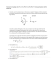### PHYSQ 126, Quiz 6 (19 mars 2015) Interaction of a Current Loop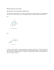### Problem Set 08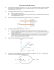### Ph 632 Problem Set #4 - Department of Physics | Oregon State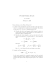### Homework #8: Magnetic Force and Biot-Savart Law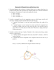### Medizinische Austellung A4 Wien 2008.indd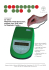### Problem Set 08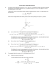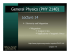### Formula sheet C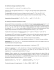### ME-709 Magnetic Recumbent Exercise Bike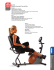### Physics 227 – Hourly Exam 2 ARC-103 (Aaa-Gzz), PLH (Haa-Lzz),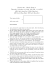### PHYS1250 Fundamental Physics Assignment Three Due Date: 5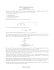### NATIONAL UNIVERSITY OF SINGAPORE DEPARTMENT OF PHYSICS ADVANCED PLACEMENT TEST (SAMPLE)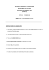### Micromag Datasheet - Eclipse Magnetics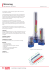### Are You Stuck on 3D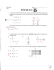### GEORGIIKOBOLD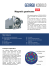### Homework #8: Magnetic Force and Biot-Savart Law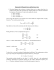### Magnetic Fields VI Name: ____________________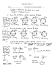### Document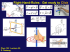### Ampere`s Law